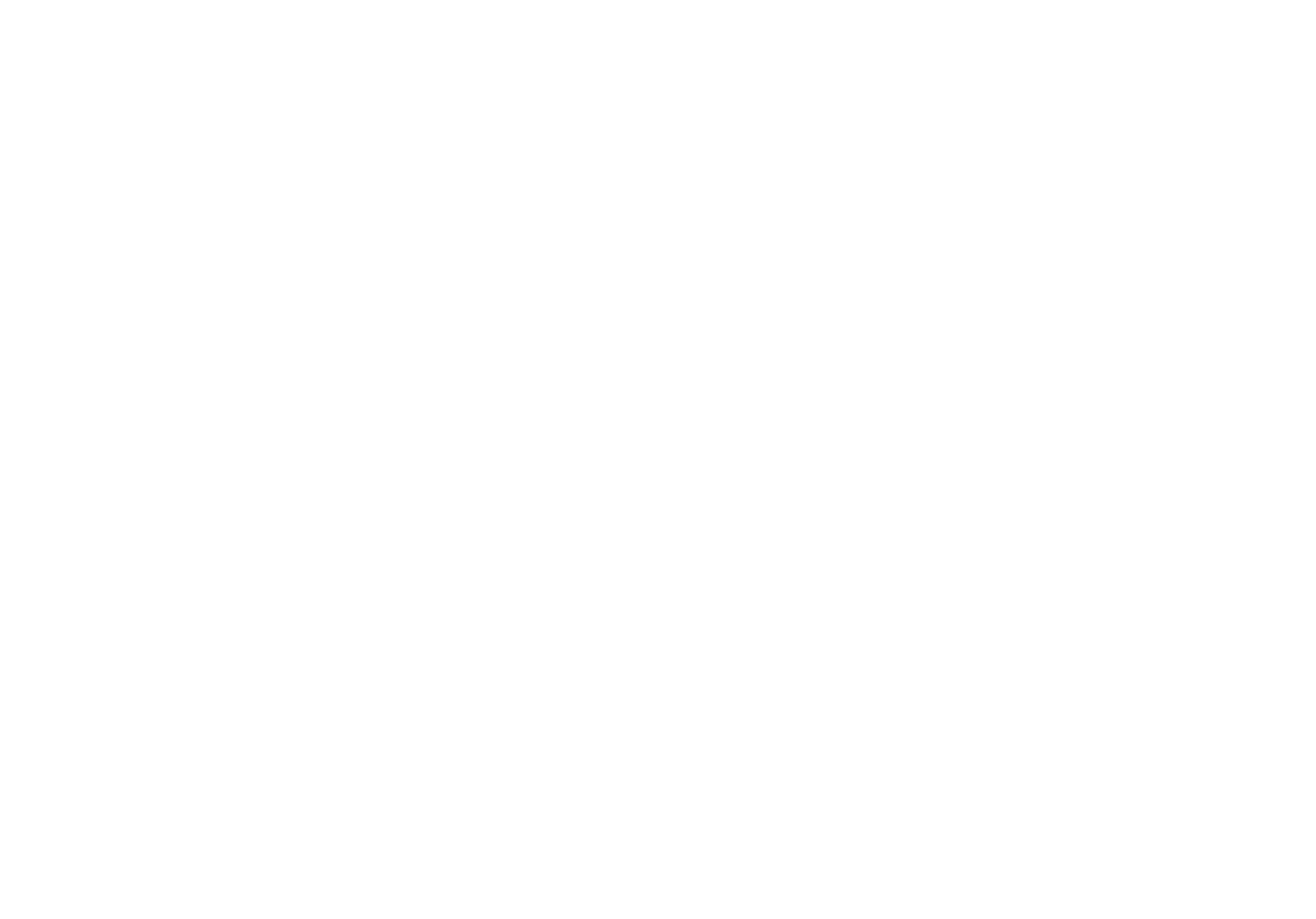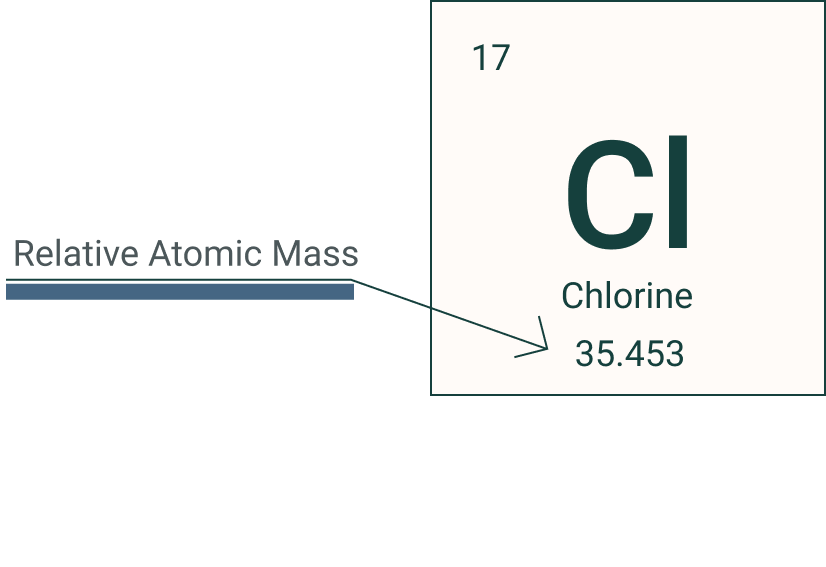Learn# Isotopes of Elements

Beginner
2
m### Isotopes are elements with different numbers of neutrons in the nucleus but the same number of protons.

Sometimes the Atomic Mass, unlike the Atomic number, is usually given as decimals. For example with Chlorine, the Atomic Mass is given as 35.5, this is because there are two forms of Chlorine as an element. This is because in nature there there are two types of Chlorine atoms and they have different numbers of neutrons but the same number of protons. As they have the same number of protons they will have the same atomic number and the same number of electrons. They have the same reactivity due to the number of electrons and also the same atomic number. The key difference is the difference in mass number, this is caused by different number of protons.  They are chemically the same, the only difference is Chlorine 35 contains 18 neutrons and Chlorine 37 contains 20 neutrons, both still have the same number of protons 17 and 17 electrons as you can see in the table.

Looking at another example Lithium has many isotopes. It has a mass number of 6.941 which in most periodic tables is rounded up to 7. This is because Lithium has isotopes that range from having 0 neutrons up to 13 but they all have different abundances. The abundance of an isotopes is the percentage of that isotope in existence. 92% of all lithium in the known universe is Lithium 7 - having 3 protons and 4 neutrons.

The relative atomic mass of an element takes into account all its naturally occurring isotopes when compared to 1/12th the mass of carbon 12. It can be mathematically calculated using the formula below.

If we look at the example of chlorine the equation would consider the abundance as a percent of the isotope mass. For Cl 35 there is 75% and for Cl 37 there is 25% so if we put this into our equation

Relative atomic mass = (35x75) + (37 x 25)

•        100

This gives a relative atomic mass of 35.5 as found in the periodic table.

###### Terms in section
Proton

A proton is a positive particle that makes up the atom in the nucleus with a positive charge

Neutron

A neutron is a neutral sub atomic particle that makes up the nucleus with the proton

Atomic mass

Atomic mass is the sum of the number of protons and neutrons in the nucleus

Atomic number

Atomic number is the number of protons in the nucleus

Electron

The electron is the smallest sub atomic particle that make up the atom. Has a negative charge and is located in shells that orbit the nucleus

Abundance

Abundance is the amount of a substance usually represented as a percentage

Relative atomic mass

Relative atomic mass is the mass of all isotopes compared to the mass of 1/12th mass of carbon 12